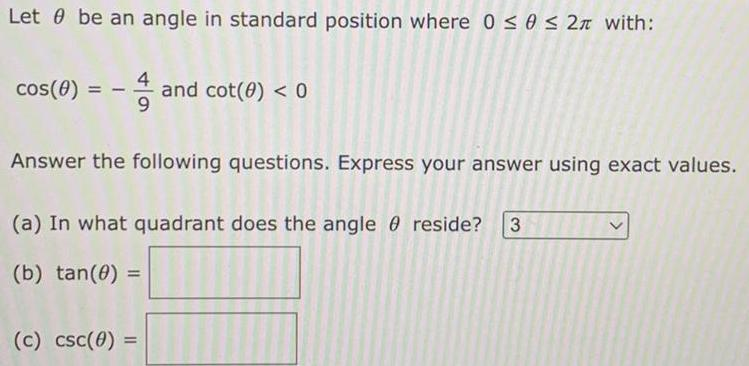Question:

# Let be an angle in standard position where 0 ≤ 0 ≤ 2л with:

Last updated: 8/12/2022Let be an angle in standard position where 0 ≤ 0 ≤ 2л with: cos(0) = -and cot(0) < 0 Answer the following questions. Express your answer using exact values. (a) In what quadrant does the angle reside? 3 (b) tan(0) = (c) csc(0) =